## 多伦多大学提出注意式吸引器网络，实现渐进式少量次学习

11 月 3 日 机器之心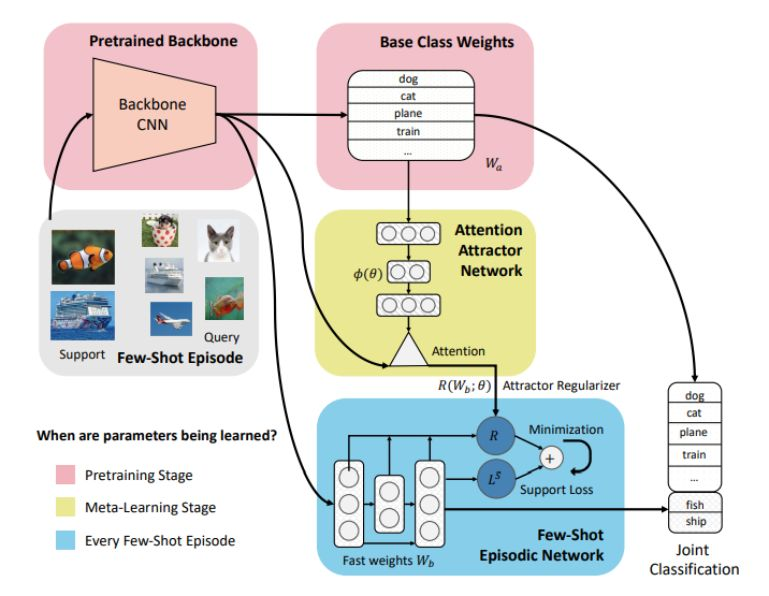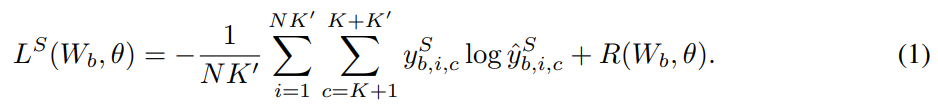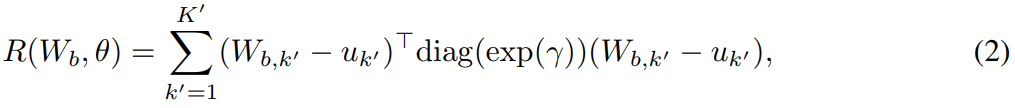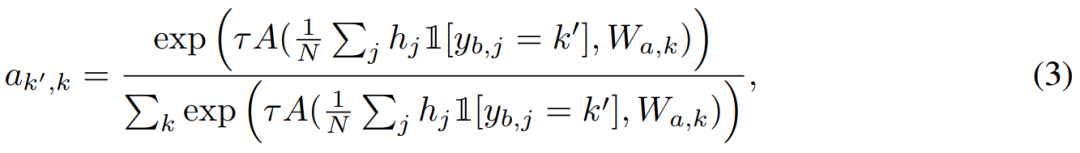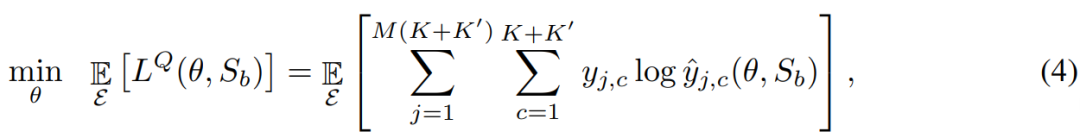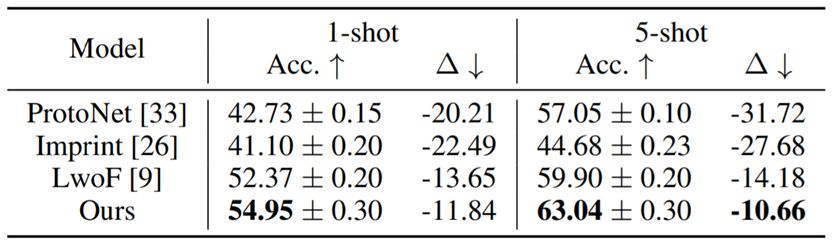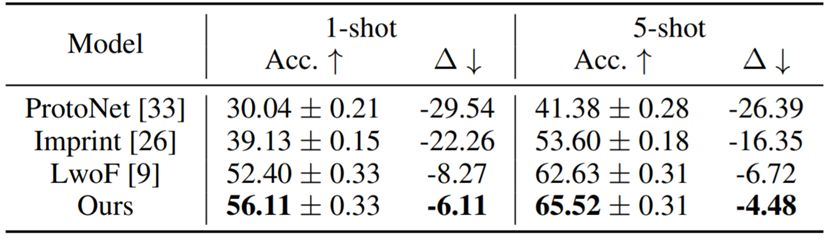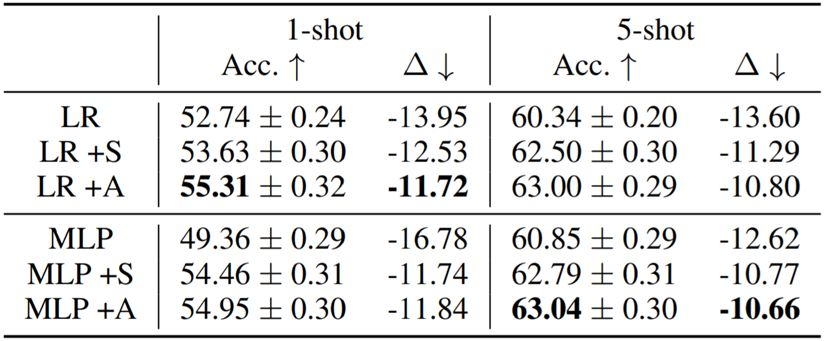表 4： 在 mini-ImageNet 上的消融实验； +S 表示静态吸引器，+A 表示注意式吸引器。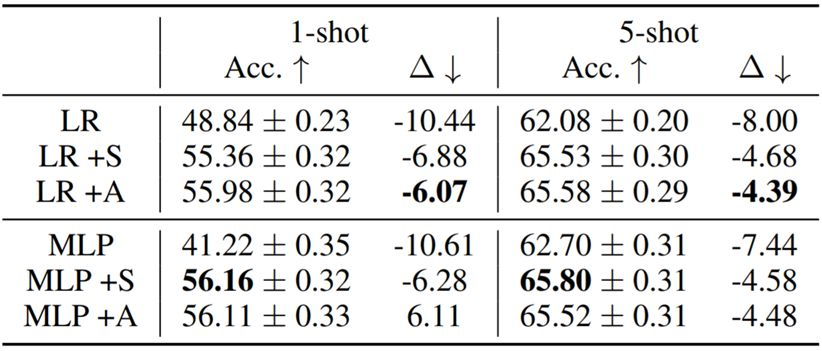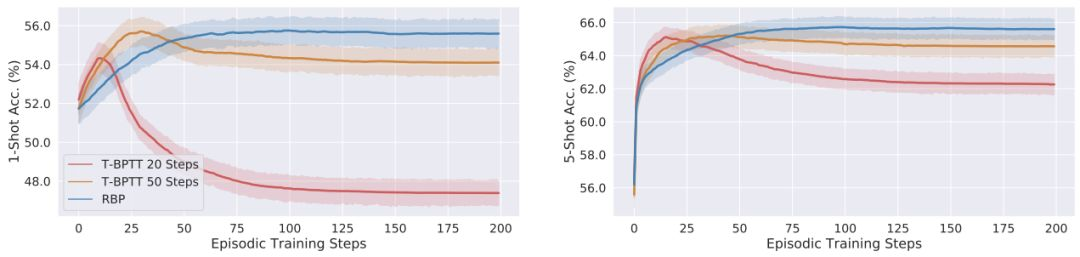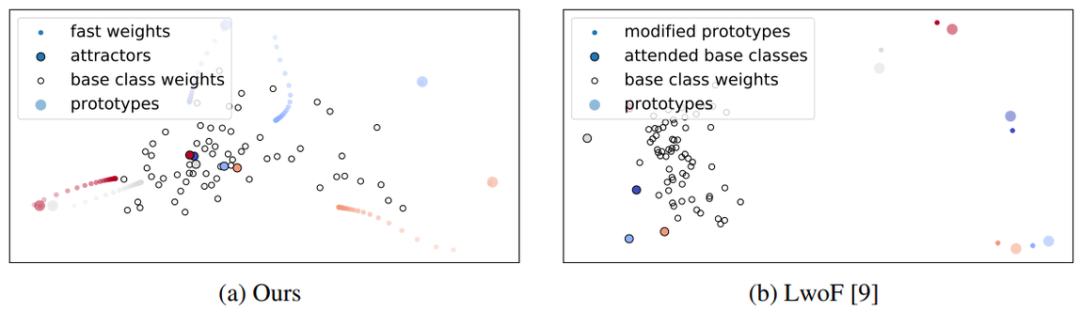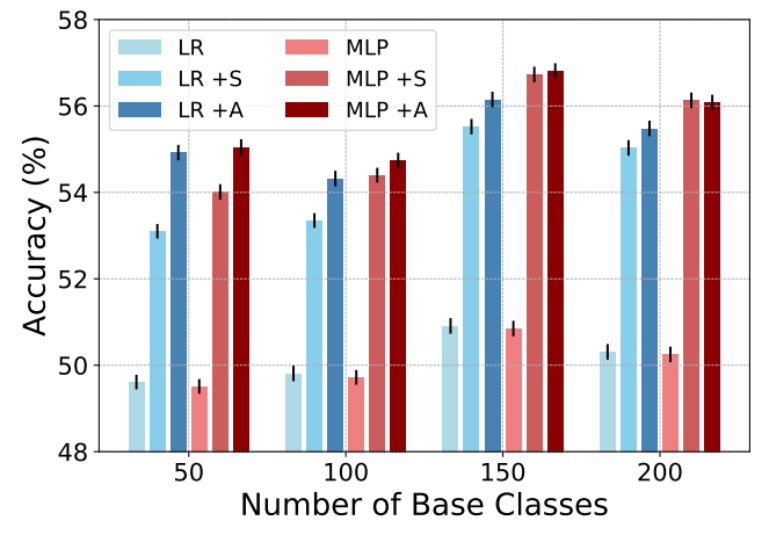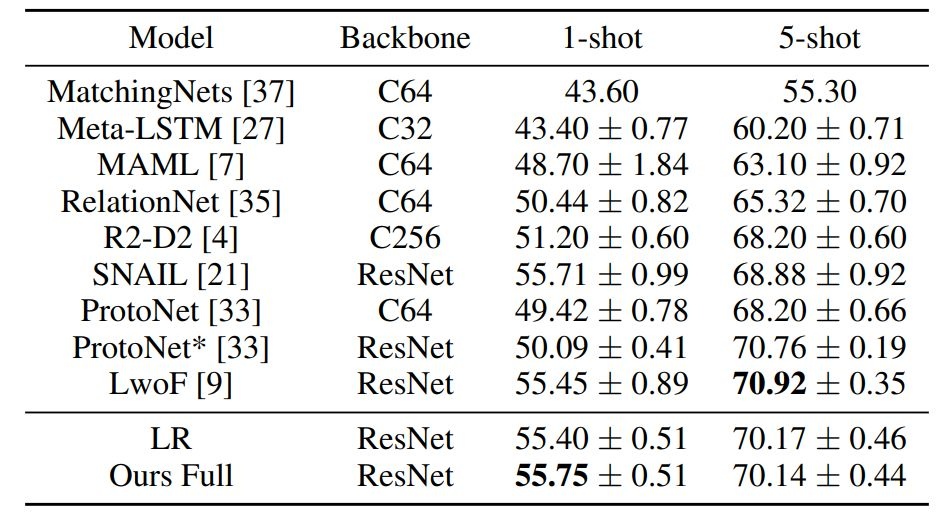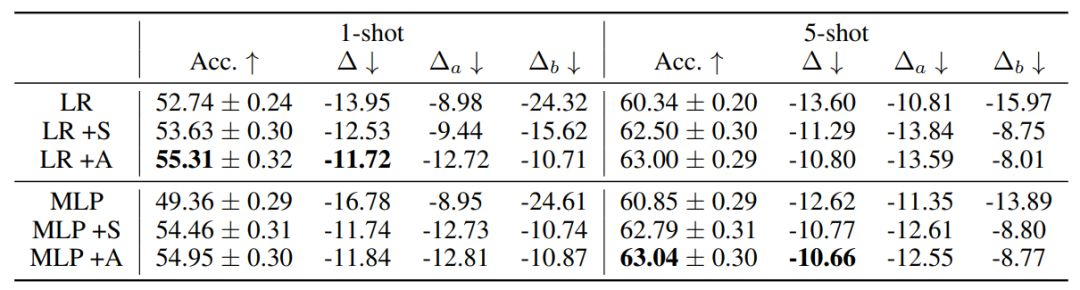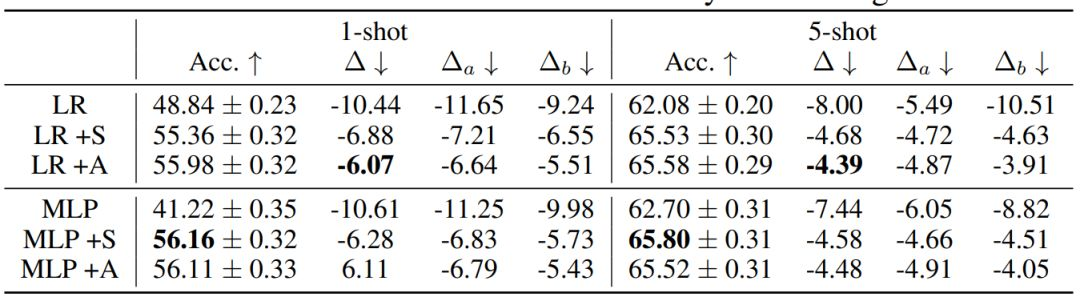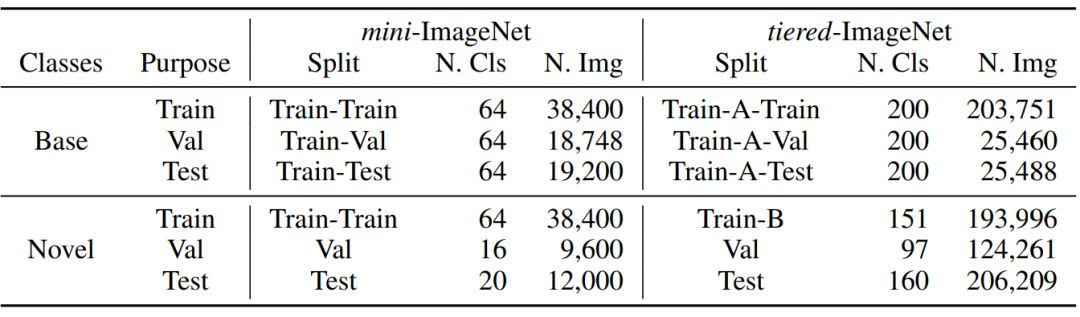✄------------------------------------------------0+3+

### 相关内容1+0+0+0+4+0+0+19+0+0+1+0+0+7+0+0+2+0+1+46+0+0+0+0+1+8+0+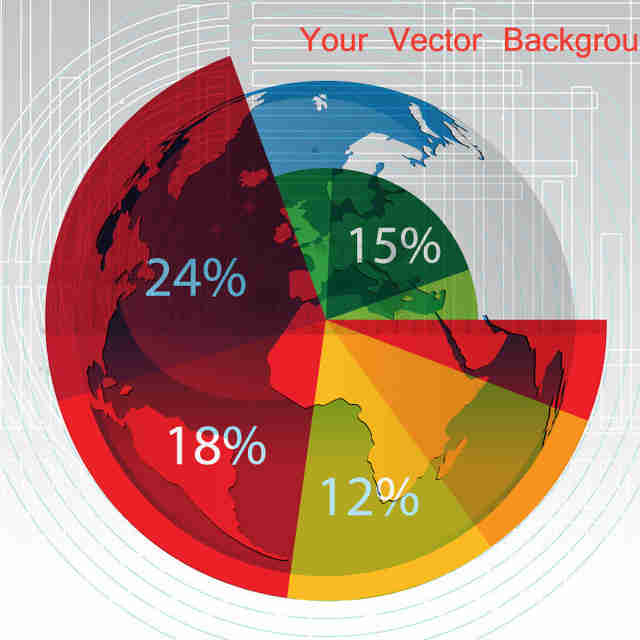0+0+0+
Top